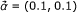1. Simplified example of position- and subtype-wise nucleotide frequencies of HIV. For three sites the subtype-wise nucleotide frequencies for subtypes A, B, C, and D are given on the left side of the table. Below them the emission probabilities estimated on the basis of only on the frequencies of the respective subtypes (using) are shown. The different typefaces (regular, bold, italic) indicate which subtypes should be jointly modelled (i.e. belong to the same source). On the right-hand side of the table, the nucleotide frequencies of the sources (i.e. the aggregated frequencies of the subtypes belonging to it) and the emission probabilities estimated on the basis of these frequencies are given (using the same). For the sake of simplicity, only the nucleotides G and T are assumed to exist. Apart from this simplification and the restriction to 4 subtypes, the example is taken from actual HIV-1 sequences.